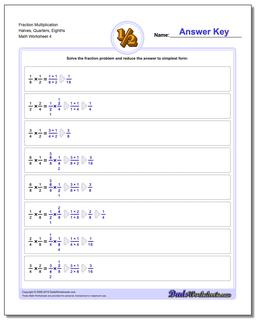# Math Worksheets: Fraction Multiplication: Fraction Multiplication: Fraction Multiplication Halves, Quarters, Eighths (Fourth Worksheet)## Fraction Multiplication Halves, Quarters, Eighths (Fourth Worksheet)

PropertyValue
DescriptionFraction Multiplication Halves, Quarters, Eighths: Basic fraction multiplication, no whole parts or cross-cancelling (Fourth Worksheet)
Resource TypeWorksheet